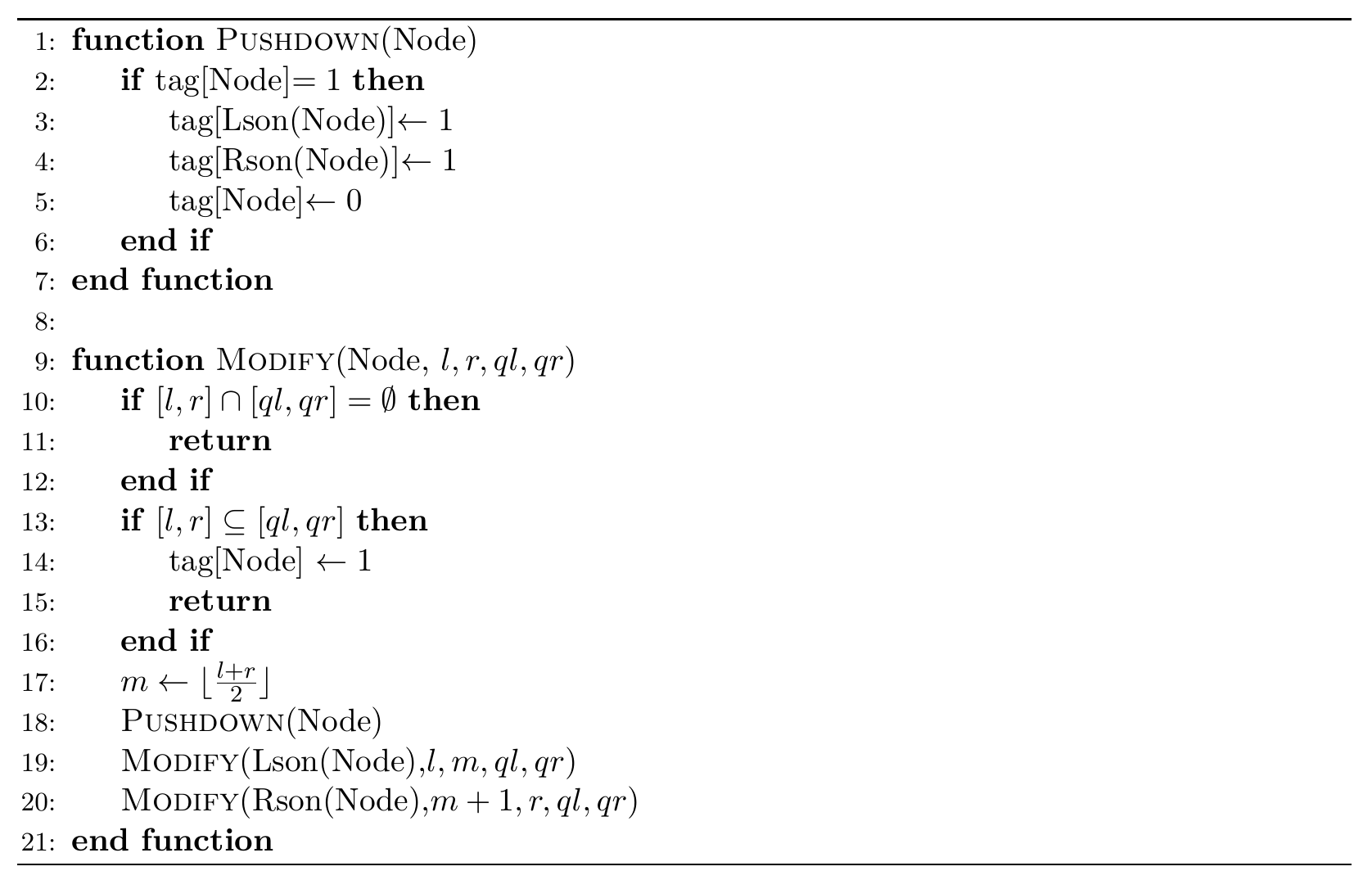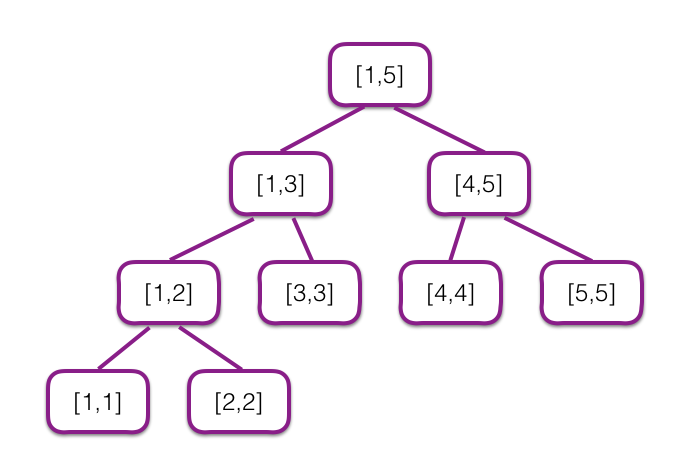# #467. 【ZJOI2019】线段树• $1\ l\ r$，假设可怜当前手上有 $t$ 棵线段树，可怜会把每棵线段树复制两份（tag 数组也一起复制），原先编号为 $i$ 的线段树复制得到的两棵编号为 $2i-1$ 与 $2i$，在复制结束后，可怜手上一共有 $2t$ 棵线段树。接着，可怜会对所有编号为奇数的线段树进行一次 $\text{Modify}(\text{root},1,n,l, r)$。
• $2$，可怜定义一棵线段树的权值为它上面有多少个节点 tag 为 $1$。可怜想要知道她手上所有线段树的权值和是多少。

### 样例一

#### input

5 5
2
1 1 3
2
1 3 5
2


#### output

0
1
6


#### explanation

$[1,5]$ 上的线段树如下图所示：• 点 $[1,3]$ 上有标记，权值为 $1$。
• 没有点有标记，权值为 $0$。

• 点 $[1,2],[3,3],[4,5]$ 上有标记，权值为 $3$。
• 点 $[1,3]$ 上有标记，权值为 $1$。
• 点 $[3,3],[4,5]$ 上有标记，权值为 $2$。
• 没有点有标记，权值为 $0$。

### 限制与约定

1 $\leq 1000$ $\leq 10$
2
3 $\leq 1000$
4
5 $\leq 10^5$ $\leq 10^5$ 询问只有一个
6
7
8
9
10